Courses

# Long Answer Type Questions- Linear Equations in Two Variables Class 9 Notes | EduRev

## Class 9 Mathematics by Full Circle

Created by: Full Circle

## Class 9 : Long Answer Type Questions- Linear Equations in Two Variables Class 9 Notes | EduRev

The document Long Answer Type Questions- Linear Equations in Two Variables Class 9 Notes | EduRev is a part of the Class 9 Course Class 9 Mathematics by Full Circle.
All you need of Class 9 at this link: Class 9

Question 1. The following observed values of x and y are thought to satisfy a linear equation.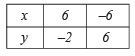Draw the graph using the values of x and y as given in the above table.
At what points the graph of the linear equation cuts the x-axis?

Solution. Plotting the points (6, –2) and (–6, 6) and joining them we get the graph AB which is the required graph.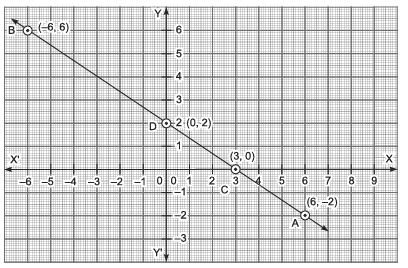The graph AB, cuts the x-axis at the point C (3, 0) and the y-axis at the point D (0, 2).

Question 2. The taxi fare in a town is 10 for the first kilometre and  6 per km for the subsequent distance. Taking the distance as ‘x’ km and total fare as y, write a linear equation for this information, what will be the total fare for 15 km?
Solution:

∵ Total distance is x km.
Total fare = ₹y
∴ x = 1 + (x – 1) = First km + Subsequent distance
Since, fare the first km = ₹10
∴ Fare for the remaining distance = ₹6 x (x – 1) = ₹6x – ₹6
⇒ Total fare = ₹10 + ₹6x – ₹6
= ₹4 + ₹6x
∴ y = 4 + 6x

⇒ y – 6x = 4
⇒ 6x – y + 4 = 0
Which is the required equation.

Now, total fare for 15 km:
6 x 15 – y + 4 = 0   [Substituting x = 15]
⇒ 90 – y + 4 = 0
⇒ 94 – y = 0
⇒ y = 94
∴ Total fare = ₹94.

Question 3. Draw the graph of the equation x – y = 4. From the graph, find the coordinates of the point when the graph line meets the x-axis.
Solution: We have x – y = 4 or y = x – 4
When x = 0, then y = 0 – 4 = –4
When x = 1, then y = 1 – 4 = –3
When x = –1, then y = – 1 – 4 =  – 5
We get the following table of values of x and y.

 x 0 1 -1 y -4 -3 -5 (x, y) (0, –4) (1, –3) (–1, –5)

∴ We have the ordered pairs of solution for x – y = 4 as (0, –4), (1, –3) and (–1, –5).
Now, plotting the points (0, –4), (1, –3) and (–1, –5) and then joining them, we get the following graph of x – y = 4.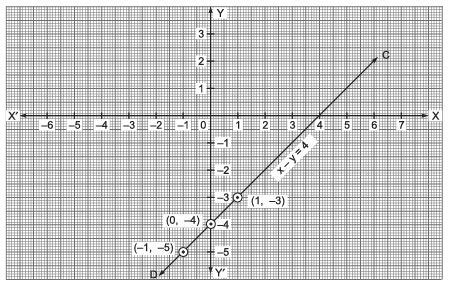From the graph, we find that the graph line meets the x-axis at (4, 0).

Question 4. Draw the graph x + 2y = 6 and from the graph, find the value of x when y = – 3.
Solution: We have: x + 2y = 6
⇒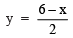When x = 0, then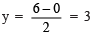When x = 2, then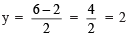When x = 4, then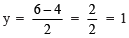We get the following table of values of x and y.

 x 0 2 4 y 3 2 1 (x, y) (0, 3) (2, 2) (4, 1)

Plotting the ordered pairs (0, 3), (2, 2) and (4, 1) and then joining them, we get the graph of x + 2y = 6 as shown below: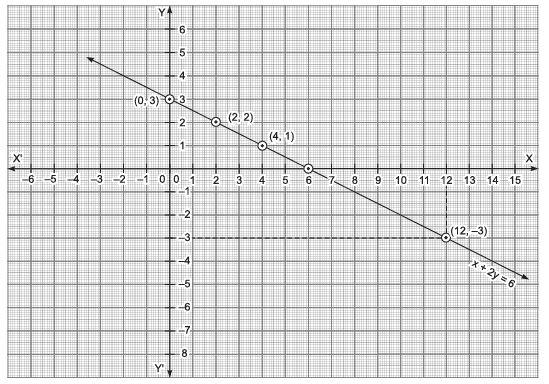From the graph, we find that for y = – 3, the value of x = 12.

132 docs

,

,

,

,

,

,

,

,

,

,

,

,

,

,

,

,

,

,

,

,

,

;